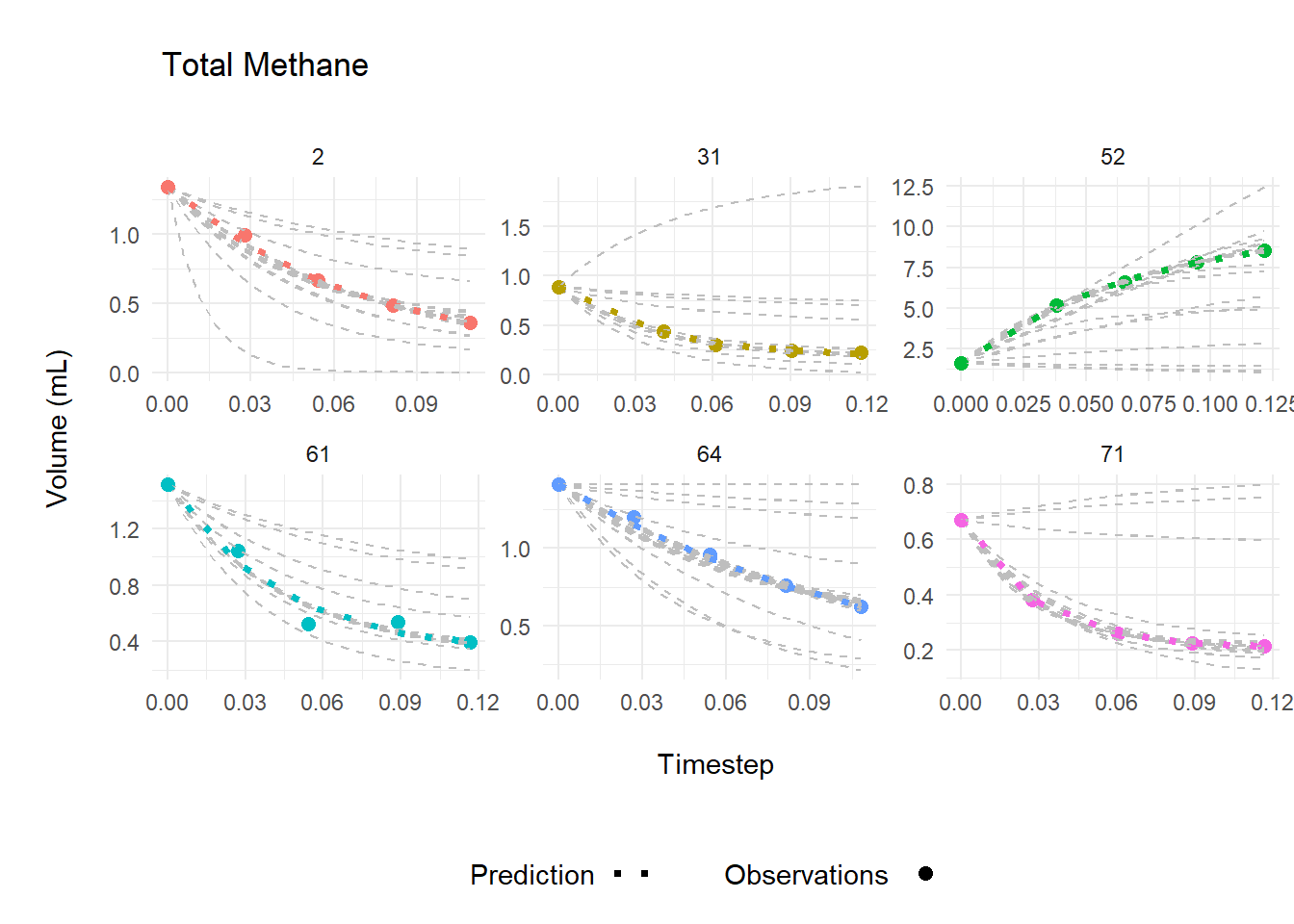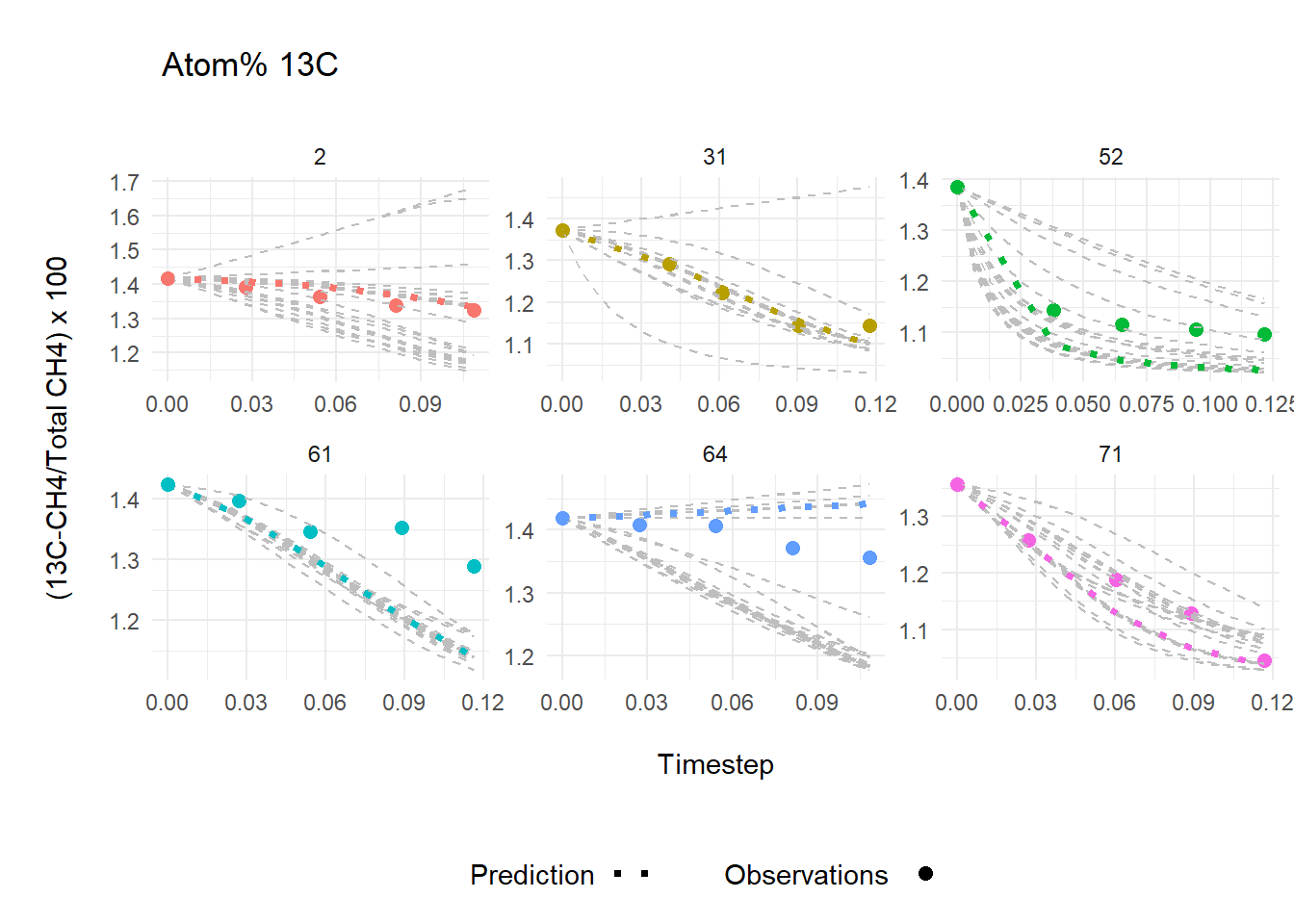# PoolDilutionR

An R package for easy calculation of rates from isotope pool dilution experiments.

To load the package, type `library(PoolDilutionR)`.

## Overview

This code calculates gross production and consumption from pool dilution time series data. Target pool size (e.g., methane, nitrate) and isotopic composition (e.g., 13C, 15N) are measured from a closed system over time, the model optimizes production rate (P) and the first order rate constant (k), by minimizing error in the model-predicted total pool size (unit volume or mass), as well as the isotopic signature. The optimization weighs the signal:noise ratio of pool and signatures using measurement precision as well as the magnitude of change over time. The calculations used here are based on von Fischer and Hedin 2002, 10.1029/2001GB001448, with some modifications (manuscript link pending).

Original code was written for data collected on a Picarro CRDS equipped with a SSIM2 unit.

## Inputs

Time series data of pool and isotopic composition.

## Functions

Prediction of P, gross production rate, and k, first order rate constant for consumption.

```````pred <- pdr_predict(time = dat\$time_days,
m0 = dat\$cal12CH4ml + dat\$cal13CH4ml,
n0 = dat\$cal13CH4ml,
P = P,
k = result\$par["k"],
pool = "CH4")```````

Optimization of predictions using data quality and deviation in pool size (Nm) and isotopic composition (Nd) over time.

```````result <- pdr_optimize(time = dat\$time_days,
m = dat\$cal12CH4ml + dat\$cal13CH4ml,
n = dat\$cal13CH4ml,
Nm = dat\$Nm,
Nd = dat\$Nd,
pool = "CH4",
params = params)`
``````

## Output

Optimized rates based on total pool size and atom percent composition.